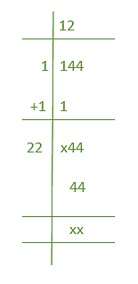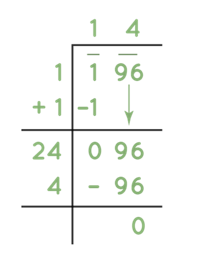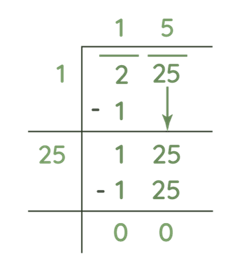GeeksforGeeks App
Open AppBrowser
Continue

# How to Find Square Root of a Number?

In everyday situations, the challenge of calculating the square root of a number is faced. What if one doesn’t have access to a calculator or any other gadget? It can be done with old-fashioned paper and pencil in a long division style. Yes, there are a variety of ways to do so. Let’s start with discussing Square root and its properties first.

### What is a Square Root?

A square root is a value, which gives the original number that multiplication of itself. e.g., 6 multiplied by itself gives 36 (i.e. 6 × 6 = 36), therefore, 6 is the square root of 36 or in other words, 36 is the square number of 6.

Suppose, a is the square root of b, then it is represented as,

a = √b or

a2 = b

Let the square of 2 is 4 so the square root of 4 will be 2 i.e.

√4 = 2

The following are square roots of the first 50 digits as:

Hence, the square root of the square of a positive number gives the original number. However, the square root of a negative number represents a complex number.

### Properties of Square Root

• A perfect square root always exists if a number is a perfect square.
• The square root of 4 is 2 and the square root of 16 is 4. So we can conclude that the square root of an even perfect square is even.
• The square root of 9 is 3 and the square root of 81 is 9. So we can conclude that the square root of an odd perfect square is odd.
• A perfect square cannot be negative and hence the square root of a negative number is not defined.
• From the above point, it can be concluded that numbers ending with (having unit’s digit) 1, 4, 5, 6, or 9 will have a square root.
• If a number of ends with an even number of zeros (0’s), then it can have a square root.
• If the unit digit of a number is 2, 3, 7, or 8 then a perfect square root is not possible.
• If a number of ends with 2, 3, 7, or 8 (in the unit digit), then the perfect square root does not exist.
• The two square root values can be multiplied. For example, √5 can be multiplied by √2, then the result should be √10.
• Two same square roots are multiplied to give a non-square root number. When √5 is multiplied by √5 we get 5 as a result.

### Perfect Square

A number that can be expressed as the product of two identical integers is called a perfect square. Perfect squares are numbers that can be made by squaring any whole number.

e.g.:

9 is a perfect square because it is the product of two equal integers, 3 × 3 = 9.

However, 10 is not a perfect square because it cannot be expressed as the product of two equal integers. (5 × 2 = 10).

Thus, a perfect square is an integer that is the square of an integer; in other words, it is the product of some integer with itself.

The numbers that are perfect squares are mentioned below, and finding the square roots of those numbers is easy. Here are few examples of square roots:

• 12 = 1
• 22 = 4
• 32 = 9
• 42 = 16
• 52 = 25
• 62 = 36
• 72 = 49
• 82 = 64
• 92 = 81
• 102 = 100

As a result, the complete squares are 1, 4, 9, 16, 25, 36, 49, 64, 81, and 100.

### Methods to find Square Root of a number

To determine if a given number is a perfect square or an imperfect square, we must first determine if it is a perfect square or an imperfect square. If the number is a perfect square, such as 4, 9, 16, etc., we will use the prime factorization process to factorize it. We must use the long division approach to find the root if the number is an incomplete square, such as 2, 3, 5, and so on.

1. Repeated Subtraction Method
2. Prime Factorization Method
3. Division Method

### 1.  Repeated Subtraction Method

The sum of the first n odd natural numbers is known to be n2. We’ll do this to calculate the square root of an integer by subtracting it several times. Let’s consider an example and see how this approach works. Let’s say you ought to find the square root of 25, which is √25. The steps are as follows:

Let’s consider the following examples to understand the repeated subtraction method to determine the square roots.

Example 1: Determine the square root of 25 using the repeated subtraction method.
Solution:

Since, 25 is an odd number. Therefore, the steps to find the square root of 25 is:

• 25 – 1 = 24
• 24 – 3 = 21
• 21 – 5 = 16
• 16- 7 = 9
• 9 – 9 = 0

Here it takes five steps to get the 0.

Hence, the square root of 25 is 5.

Example 2: Determine the square root of 16 using the repeated subtraction method.
Solution:

Since, 16 is an even number. Therefore, the steps to find the square root of 16 is:

• 16 – 4 = 12
• 12 – 4 = 8
• 8 – 4 = 4

Here it takes four steps to get the 0.

Hence, the square root of 16 is 4.

Example 3: Find the square root of 49 using the repeated subtraction method.

Solution:

Since, 49 is an odd number. Therefore, the steps to find the square root of 49 is:

• 49 – 1 = 48
• 48 – 3 = 45
• 45 – 5 = 40
• 40 – 7 = 33
• 33 – 9 = 24
• 24 – 11 = 13
• 13 -13 = 0

Here it takes seven steps to get the 0.

Hence, the square root of 49 is 7.

### 2.  Prime Factorization Method

The prime factorization method involves expressing numbers as a function of their prime factors. The square root of the number is given by the product of one element from each pair of equal prime factors. This approach can also be used to determine whether a given number is a perfect square or not. This method, however, cannot be used to find the square root of non-perfect square decimal numbers.

e.g.: The prime factors of 126 will be 2, 3 and 7 as 2 × 3 × 3 × 7 = 126 and 2, 3, 7 are prime numbers.

• 16 = 2 × 2 × 2 × 2 = 22 × 22 = √16 = 4
• 25 = 5 × 5 = 52 = √25 = 5
• 64 = 2 × 2 × 2 × 2 × 2 × 2 = √64 = 8

### 3.  Division Method

When the integers are sufficiently large, it is easy to obtain the square root of a perfect square by utilizing the long division approach, because getting their square roots through factorization becomes lengthy and complicated. To overcome this problem, a new method for finding the square root is developed. This method basically uses the division operation by a divisor whose square is either less than or equal to the dividend.

Following are the steps to for division method:

Step 1: Take the number whose square root is to find. Place a bar over every pair of the digit of the number starting from that in the unit’s place (rightmost side).

Step 2: Let’s divide the leftmost number by the largest number whose square is less than or equal to the number under the leftmost bar. Take this number as the divisor and the quotient. The number under the leftmost bar is considered to be the dividend.

Step 3: Divide and get the number. Bring down the number under the next bar to the right of the remainder.

Step 4: Double the divisor (or add the divisor to itself). To the right of this divisor find a suitable number which together with the divisor forms a new divisor for the new dividend. The new number in the quotient will have the same number as selected in the divisor. The condition is the same as being either less or equal to that of the dividend.

Step 5: Continue this process till we get zero as the remainder. The quotient thus obtained will be the square root of the number.

Let’s consider the following examples to understand the division method to determine the square roots.

Example 1: Find the square root of 144 using the division method.

Solution:

The steps to determine the square root of 144 are:

Step 1: Start the division from the leftmost side. Here 1 is the number whose square is 1.

Step 2: Putting it in the divisor and the quotient and then doubling it will give as,Step 3: Now it is required to find a number for the blanks in divisor and quotient. Let that number be x.

Step 4: Therefore, check when 2x multiplies by x give a number of less than or equal to 44. Take x = 1, 2, 3, and so on and check.

In this case,

• 21 × 1 = 21
• 22 × 2 = 44

So we choose x = 2 as the new digit to be put in the divisor and in the quotient.

The remainder here is 0 and hence 12 is the square root of 144.

Example 2: Find the square root of 196 using the division method.

Solution:

The steps to determine the square root of 196 are:

Step 1: Start the division from the leftmost side. Here 1 is the number whose square is 1.

Step 2: Putting it in the divisor and the quotient and then doubling it will give.Step 3: Now we need to find a number for the blanks in divisor and quotient. Let that number be x.

Step 4: We need to check when 2x multiplies by x give a number less than or equal to 96. Take x = 1, 2, 3 and so on and check.

In this case,

• 21 × 1 = 21
• 22 × 2 = 44
• 23 × 3 = 69
• 24 × 4 = 96

So, choose x = 4  as the new digit to be put in divisor and in the quotient.

The remainder here is 0 and hence 14 is the square root of 196.

Example 2: Find the square root of 225 using the division method.

Solution:

The steps to determine the square root of 225 are:

Step 1: Start the division from the leftmost side. Here 1 is the number whose square is 1.

Step 2: Putting it in the divisor and the quotient and then doubling it will give.Step 3: Now we need to find a number for the blanks in divisor and quotient. Let that number be x.

Step 4: We need to check when 2x multiplies by x gives a number which is either less than or equal to 125. Take x = 1, 2, 3 and so on and check.

In this case,

• 21 × 1 = 21
• 22 × 2 = 44
• 23 × 3 = 69
• 24 × 4 = 96
• 25 × 5 = 125

So we choose x = 5 as the new digit to be put in divisor and in the quotient.

The remainder here is 0 and hence 15 is the square root of 225.

### 4.  Square Roots of Complex Numbers

To calculate the square root of a complex number, let’s suppose that the root is ea + ib. Then compare it to the original number to get the values of a and b, yielding the square root.

Let a + ib is a complex number, therefore to find the square root of a + ib following formula can be usedLet’s consider the following examples to understand the determination of the square roots of complex numbers.

Example 1: Find the square root of 6 – 8i.

Solution:

Let’s use the following formula to determine the square root of the given complex number as:For the given case, substitute a = 6 and b = (-8) in the above formula,which is the required solution.

Example 2: Find the square root of 9 + 40i.

Solution :

Let’s use the following formula to determine the square root of the given complex number as:For the given case, substitute a = 9 and b = 40 in the above formula,which is the required solution.

Example 3: Find the square root of 3 + 4i.

Solution :

Let’s use the following formula to determine the square root of the given complex number as:For the given case, substitute a = 3 and b = 4 in the above formula,which is the required solution.

My Personal Notes arrow_drop_up
Related Tutorials